## Symmetry and the Fourth Dimension (Part 2)

### The Coxeter group of the cube

Coxeter groups are a huge amount of fun. Normally their delights are reserved for people who have already studied group theory. But I don’t think that’s fair. You don’t need to know music theory to enjoy a piece by Bach. And you don’t need to know group theory to enjoy Coxeter groups.

In fact, it’s probably better to learn theories after falling in love with some examples. Imagine a world where you had to learn music theory before listening to music. A world where everyone studied music theory in elementary school, high school and college, but only people who majored in music were allowed to listen to the stuff. In that world, people might say they hate music… just as in this world they say they hate math.

So, here goes:

Last time I showed you that any Platonic solid has a bunch of symmetries where we reflect it across planes. These planes, called mirrors, all intersect at the center of the solid. If we take a sphere and slice it with these mirrors, it gets chopped up into triangles, and we get a pattern called a Coxeter complex.

If we start with the cube, here’s the Coxeter complex we get: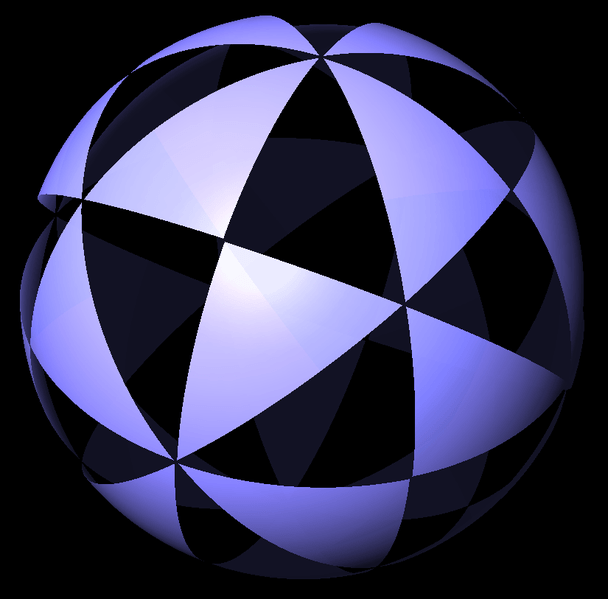For artistic reasons, half the triangles are colored blue and half are colored black. But it’s not just pretty: there’s also math here. If we take any black triangle and reflect it across any mirror, we get a blue triangle… and vice versa.

Instead of taking a sphere and slicing it with mirrors, we can start with the cube itself. Here’s what we get: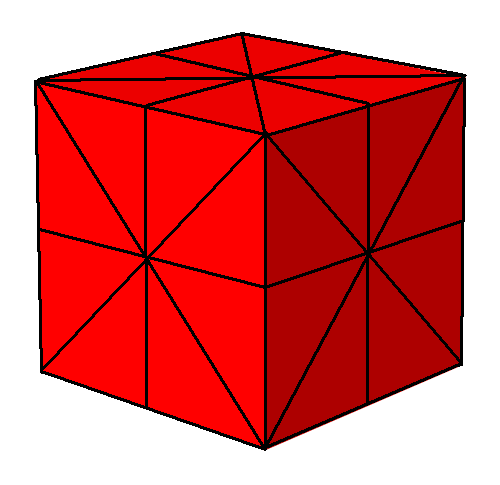It’s not quite as pretty (especially because I drew it), but it makes certain games easier to play. These games involve picking one triangle and calling it our favorite. It doesn’t matter which. But we have to pick one… so how about this:Each different symmetry of the cube sends this triangle to a different triangle. This instantly lets us count the symmetries: there are 48, since each of the cube’s 6 faces has been chopped into 8 triangles.

But even better, we get a vivid picture of the symmetries of the cube! Let’s see how this works.

Any triangle in the Coxeter complex has three corners:

• one corner is a vertex of the cube,

• one corner is the center of an edge of the cube,

• one corner is the center of a face of the cube.

Here’s how it works for our favorite triangle:Now the real fun starts. We can move from any triangle to a neighboring one in three ways:

1) We can change which vertex of the cube our triangle contains. Starting from our favorite triangle, we get this blue triangle: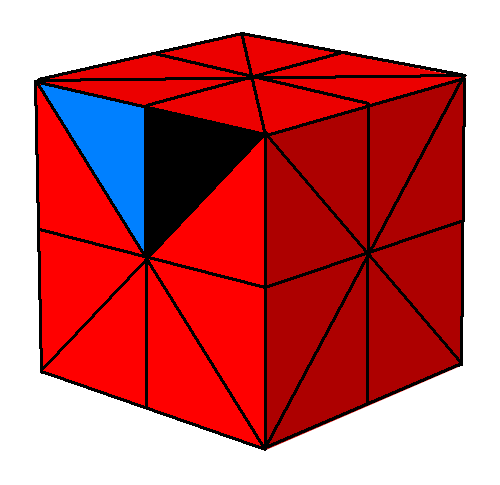Note: the blue triangle touches the same edge of the cube as the black one. It also lies on the same face. Only the vertex has changed!

What have we actually done here? We’ve reflected our triangle across a mirror in a way that changes which vertex of the cube it contains. Let’s call this way of flipping a triangle V.

2) We can change which edge of the cube our triangle touches. Starting from our favorite triangle, we get this yellow triangle: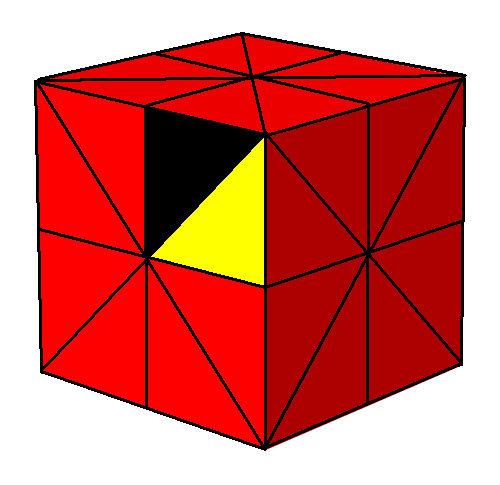Note: the yellow triangle contains the same vertex of the cube. It also lies on the same face. Only the edge has changed!

What have we actually done here? We’ve reflected our triangle across a mirror in a way that changes which edge of the cube it touches. Let’s call this way of flipping a triangle E.

3) We can change which face of the cube our triangle lies on. Starting from our favorite triangle, we get this green triangle: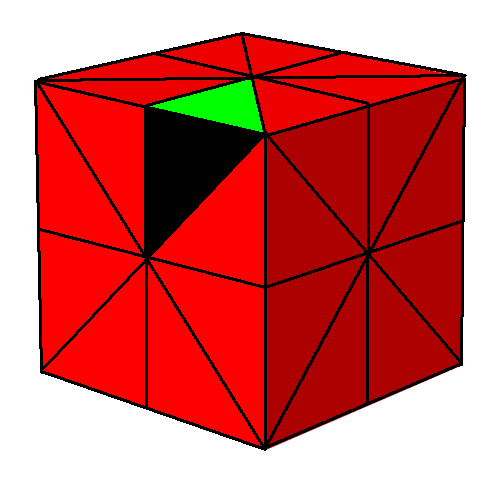Note: the green triangle contains the same vertex of the cube. It also touches the same edge. Only the face has changed.

What have we actually done here? If you can’t guess, you must be asleep: we’ve reflected our triangle across a mirror in a way that changes which face of the cube it lies on! Let’s call this way of flipping a triangle F.

By repeating these three operations—changing the vertex, edge or face—we can get to any triangle starting from our favorite one. We can even use this trick to label all the triangles. Let’s call our favorite triangle 1: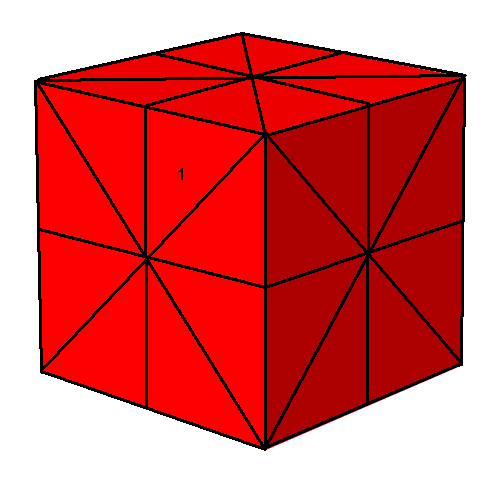Let’s call its neighbors F, E and V, since we use those three reflections to get to these new triangles:Starting with these, we can get more triangles by changing the vertex, edge or face: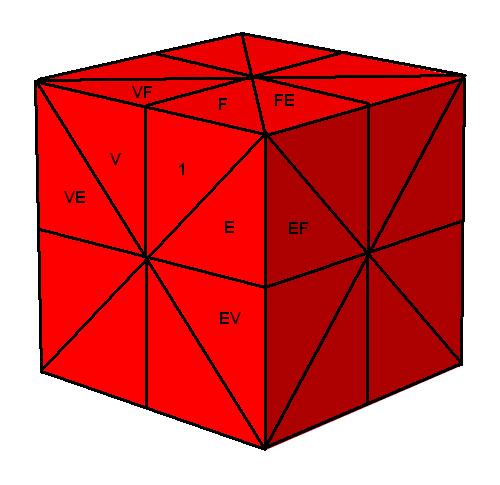See what I’m doing? We get the triangle VE by starting with the triangle V and then changing which edge of the cube it contains. We get EF by starting with E and then changing which face it lies on. And so on.

However, there’s a ‘problem’. See the triangle VF? We got there from the triangle V by changing which face it lies on. But we could also get there another way! We could start at F and then change which vertex this triangle contains. So we could equally well call this triangle FV.

Luckily, in math nothing is really a problem once you understand it. This is why math is more fun than real life: merely understanding a problem makes it go away. We’ll just say that

VF = FV

So, we can use either label for this triangle: it doesn’t matter.

More deeply, if you start with any triangle, change the vertex it contains and then change the face it lies on, you get the same triangle as if you first change the face and then the vertex. That’s what the equation VF = FV really means. It’s a fact of geometry: a general fact about Platonic solids.

Let’s go a bit further: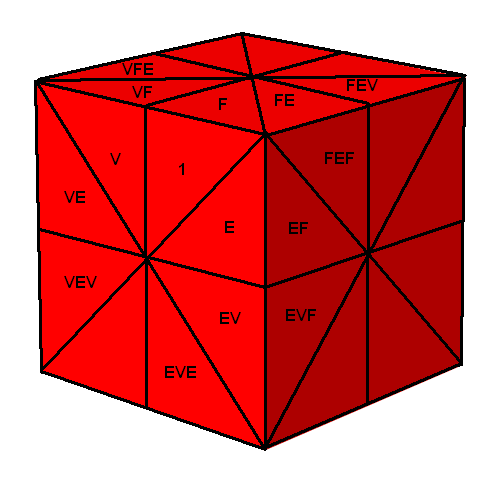I’m using the same rules; check to make sure I did everything right! There’s another little ‘problem’, though: see the triangle labelled FEF? We got there from FE by changing which face of the cube our triangle lies on. But we could also get there starting from EF by changing the edge. So really we have

FEF = EFE

But this is not a general fact about Platonic solids: it shows up because in the cube, three faces and three edges meet at each vertex. That’s why the equation has three F’s and three E’s.

We can go on even further, but you can already see where the next problem will show up. See that unlabelled triangle in the front face of the cube? At the next stage we’ll want to label it VEVE, but we’ll also want to label it EVEV. So:

VEVE = EVEV

Again, this is not a general fact about Platonic solids! It shows up because the cube has square faces, so four vertices and four edges touch each face. That’s why the equation has four V’s and four E’s.

We almost have enough equations to avoid all future problems. But there are a few more that are so obvious you may have overlooked them. Suppose we change which vertex of the cube our triangle contains, and then do this again. We get back where we started! For example, first we go from the black one to the blue one:and then we go back to black.

So, we have

VV = 1

This says switching vertices twice gets us back where we started. Similarly, we have

EE = 1

and

FF = 1

And now, although I haven’t proved it to you, we have a complete set of equations to give each triangle an unambiguous name… or more precisely, an unambiguous element of the ‘Coxeter group’ of the cube. Two different expressions, like EFE and FEF, give the same element of the Coxeter group if we can get from one to the other using our equations. For example, in the Coxeter group we have

FEFVVEFE = FEFEFE = FEFFEF = FEEF = FF = 1

### Coxeter groups of Platonic solids

We can do this stuff for other Platonic solids, too. The Coxeter group of the octahedron is secretly the same as that of the cube, since they’re dual. The only difference is that the names F and V get switched, because faces of the cube correspond to vertices of the octahedron, and vice versa! Similarly for the icosahedron and dodecahedron. So, I mainly have two puzzles for you:

Puzzle 1: Find equations defining the Coxeter group of the tetrahedron.

Puzzle 2: Find equations defining the Coxeter group of the dodecahedron.

If these seem hard, let’s reflect a bit more on what we did for the cube. For the cube we have

VF = FV

because of this picture:Similarly, we have

EFE = FEF

because of this picture:And finally, we have

VEVE = EVEV

because of this picture: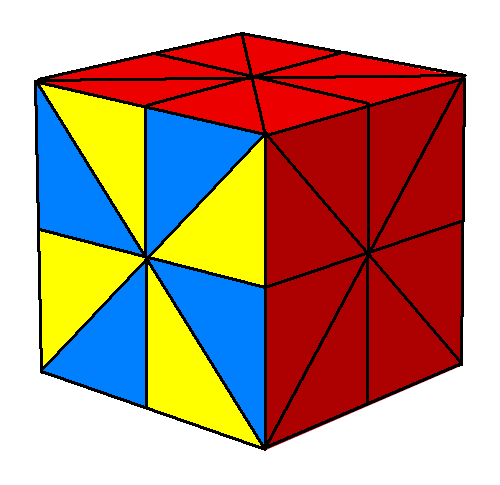This should make it easy to solve the puzzles. We can also phrase the solutions in a different way:

Puzzle 3: Show that that Coxeter groups of the tetrahedron, cube and dodecahedron can be completely described by the equations

V2 = E2 = F2 = 1

and

(VE)a = (VF)b = (EF)c = 1

for some integers a, b, and c.

The story doesn’t stop here—far from it! Later we’ll meet Coxeter groups for the higher-dimensional analogues of Platonic solids, which are called regular polytopes. And we’ll use them to classify so-called uniform polytopes obtained by chopping vertices, edges, faces and so on off the regular ones. For example, the cuboctahedron:can be gotten either by chopping the corners off a cube, or chopping the corners of an octahedron! We can classify such shapes using Coxeter diagrams, which are based on Coxeter groups. So, there’s no shortage of fun stuff to do… in fact, there’s way too much!

Actually, that’s the main problem with mathematics, once you start actually doing it. There’s just too much fun stuff.

### 18 Responses to Symmetry and the Fourth Dimension (Part 2)

1.qinglong1397 says:

Reblogged this on 公丘子 and commented:
I like it. Thanks to John Baez

2.Greg Egan says:

I’m a bit confused about the notational conventions here. On the diagrams, it looks as if you’re multiplying reflections on the left: for example, the triangle you label EF is the triangle reached by taking the triangle labelled 1, multiplying by (the reflection that takes 1 to) F, then multiplying by (the reflection that takes 1 to) E. The triangle you label FE is reached from 1 by multiplying first by (the reflection that takes 1 to) E, then multiplying by (the reflection that takes 1 to) F.

But in the text, you seem to describe the labels as if you’re multiplying on the right:

[S]ee the triangle labelled FEF? We got there from FE by changing which face of the cube our triangle lies on.

•Greg Egan says:

After re-reading this I think I understand what you’re getting at in the text: you’re describing these labels without referring to the multiplication of reflections at all, purely in terms of the incidence relations between the triangles and features of the cube.

So the triangle FEF in this scheme is defined as the triangle that intersects the same vertex and edge of the cube as FE, while intersecting a different face. That this also corresponds to the triangle reached by applying group elements F then E then F to the triangle labelled 1 is a separate fact. So I should probably try to prove that both ways of interpreting the labels are equivalent.

•Greg Egan says:

If S is any string of Es, Fs and Vs interpreted as reflections multiplied together and then applied to the triangle 1, the triangles SE, SV and SF will each bear the same relationship to the triangle S as the triangles E, V and F, respectively, bear to the triangle 1, in terms of which features of the cube they have in common. Any symmetry of the cube will preserve incidence relations, so applying S (as a symmetry) to, say, triangle 1 and triangle E will yield triangles S and SE that intersect different edges of the cube but share the same vertex and face.

So the two ways of interpreting the labels are compatible. Sorry to be thick, this is pretty obvious in retrospect.

•John Baez says:

Your first comment got me scared for a minute, because I tend to mix up left and right, and I also have a habit of tinkering with standard conventions—an explosive combination. Since I was trying to make everything easy for nonmathematicians, I wanted a convention where flipping our favorite triangle to one touching a new Edge and then to one lying on a new Face is called EF, and so on.

After re-reading this I think I understand what you’re getting at in the text: you’re describing these labels without referring to the multiplication of reflections at all, purely in terms of the incidence relations between the triangles and features of the cube.

Right.

So the triangle FEF in this scheme is defined as the triangle that intersects the same vertex and edge of the cube as FE, while intersecting a different face.

Right. Do you think there’s any way for me to make this clearer without bringing in phrases that will be mysterious to mathematicians, like ‘multiplying reflections’? I’ll probably try to turn all these posts into an article someday… Even if I’m aiming my discussion at nonmathematicians, I don’t want mathematicians to be confused by it!

That this also corresponds to the triangle reached by applying group elements F then E then F to the triangle labelled 1 is a separate fact. So I should probably try to prove that both ways of interpreting the labels are equivalent.

Indeed, this is a somewhat confusing issue, the sort I have to work out each time—just like remembering whether to do things earlier or later when ‘daylight savings time’ starts in the US… especially when phoning my mom from Singapore!

All these questions are related to the ‘active versus passive coordinate transformation’ issue that physicists love to talk about—and mathematicians who don’t take physics courses seem never to have heard of.

We can think of a chosen triangle on the cube as putting coordinates on the cube. Then we can think of doing V, E or F to the triangle as changing these coordinates while leaving the cube fixed. This is what physicists would call a ‘passive’ coordinate transformation.

There are annoying left-right issues involved in switching from the ‘active’ versus ‘passive’ conventions, since an active transformation on some space$x \mapsto g(x)$

has the following effect on functions on that space, e.g. coordinate functions:$f \mapsto f \circ g$

So, you can pile this confusion on top of any caused by my nonstandard conventions when pondering this issue.

•John Baez says:

I think I see one way I can reduce confusion: don’t say “let’s call this reflection E…”, or at least say it some better way.

•Greg Egan says:

I must confess that I didn’t read the text carefully the first time, because the diagrams alone convinced me that you were multiplying on the left with reflections called E, V and F. People coming at this post without any preconceptions wouldn’t assume that.

But since inevitably some people reading your posts will have had some prior exposure to group theory, maybe it would help them if you give a hint near the start that you’re playing a different game, even if it turns out to be isomorphic to the one they’re expecting!

What’s nice about your way of generating the labels is that it’s incredibly easy to see what they should be, whereas applying a given reflection to a triangle that doesn’t even touch the reflection plane takes a lot more effort.

•John Baez says:

Thanks—yes, I think this ‘triangle-flipping’ approach makes the whole subject easier. It’s not really new to me: it’s implicit in the theory of Coxeter complexes, buried under layers of jargon.

In this approach, the triangulated cube is the symmetry group of the cube! In other words: once we’ve chosen our favorite triangle, each triangle is named by the group element that carries our favorite triangle to that one.

This made me think about how the 2-sphere can’t be made into a Lie group. The triangulated cube seems like a ‘good attempt at solving an impossible problem’, since the surface of a cube is topologically a 2-sphere, and we’ve made the set of triangles into a group. The group operation is even ‘continuous’ in some funny sense: triangles touching the identity (our favorite triangle) act as operations that move a triangle to one that touches it! Alas, I don’t know what concept of ‘continuity’ this is an example of.

Actually, the triangulated dodecahedron is an even better attempt, in the sense that we get more triangles.

•Mike Stay says:

The rotation subgroup is also continuous, since a pair of reflections leaves one vertex in common. It’s also a subgroup of SO(3).

•John Baez says:

I don’t really get what you mean, Mike. Of course the rotational symmetries of anything 3-dimensional form a subgroup of SO(3), and yes, a rotational symmetry of a cube leaves an antipodal pair of these triangle corners fixed. But I don’t know what you mean by “the rotation group is also continuous”.

•Mike Stay says:

I just meant that it’s continuous in your “funny sense”, and rotations seem more amenable to becoming a Lie group than reflections.

•John Baez says:

I don’t see how to subdivide the cube’s surface into polygons, one for each of its 24 rotational symmetries, such that the symmetries corresponding to the polygons touching the polygon for the identity act to map any other polygon to one that touches it. You seem to be hinting that you can do it.

I _can_ do it for rotational/reflectional symmetries of the cube.

Some relevant bits of topology:

The rotation group of Euclidean 3-space is SO(3), which is topologically the projective 3-space$\mathbb{R}P^3.$ The rotation/reflection group of Euclidean 3-space is O(3), which is topologically two disjoint copies of the projective 3-space$\mathbb{R}P^3.$

Jim Stasheff proved some very general theorems saying stuff like: there’s no way to make any space that’s homotopy equivalent to$S^2$ into a topological group.

3.John Baez says:

Over on G+, Shanthanu Bhardwaj has solved the puzzles. Click here and read his comment if you want your fun to be spoiled by seeing the solutions.

4. […] John Baez wants you to fall in love with the Coxeter group of the cube […]

5.Daniel says:

Dear Sir,
I was really inspired and overwhelmed by the website you and your colleagues run (http://math.ucr.edu/home/baez/README.html). I think that it is really noble of you to just let the general public access the.pdf files of your books for free and to offer your valuable advice at no cost, when you could have charged them for it. I wish you and your colleagues all the best, and once again, thank you so much for taking your valuable time to maintain and update this blog and the above website.

•John Baez says:

Thanks! I didn’t go into mathematics to make money, and in fact I’m a lot more well-off than I ever expected to be. Given all that, it seems ridiculous to charge money for my books… especially since these books never made much money in the first place, and illegal Russian websites offer most scientific books for free anyway.

But anyway, I’m glad you’re enjoying all the material on that website.

6. […] in Part 2, we looked at operations that flip these triangles around. I showed you how it works for the cube. […]

7.Emi says:

Reblogged this on Pathological Handwaving and commented:

2!!!

This site uses Akismet to reduce spam. Learn how your comment data is processed.